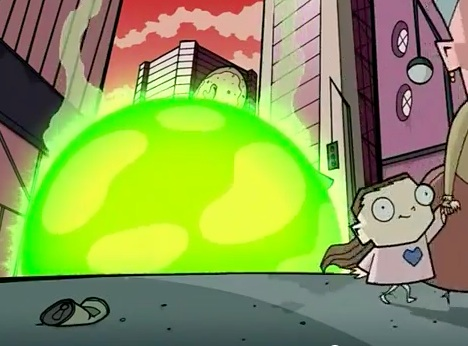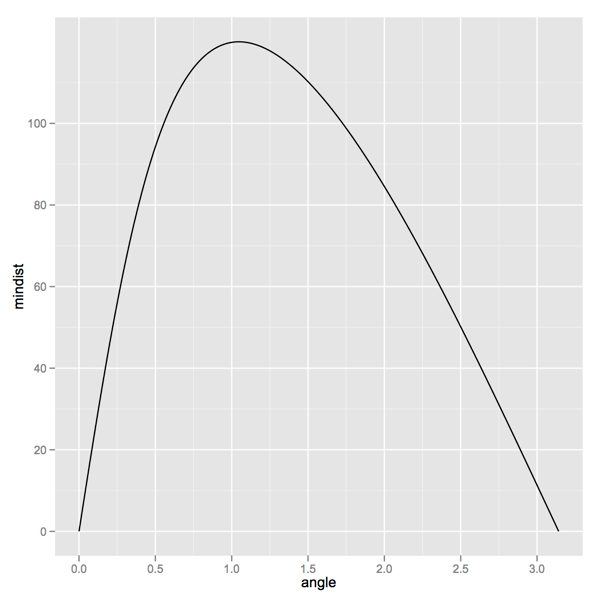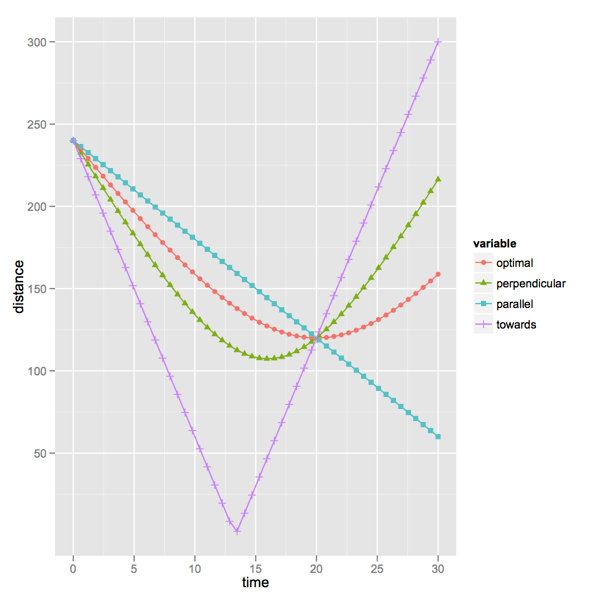# How to outrun a crashing alien spaceship

Hollywood movies are obsessed with outrunning explosions and outrunning crashing alien spaceships. For explosions the movies give the optimal (but unusable) solution: run straight away. For crashing alien spaceships they give the same advice, but in this case it is wrong. We demonstrate the correct angle to flee.Running from a crashing alien spaceship, Prometheus 2012, copyright 20th Century FoxTo flee an explosion the movies give the right advice: run straight away. They incorrectly give the impression that you can outrun a nearby explosion that expands faster than you run, but the advice is optimal.Die Hard, copyright 20th Century Fox

However, for slower explosions this is useful advice.But what about outrunning a crashing alien spaceship? Since the spaceship is not expanding the situation is no longer isotropic. Some directions may be better than others. In fact if the spaceship is moving faster than you, getting out of its way may be better than trying to outrun it. So we consider the following diagram of the situation:If the crashing spaceship is approximated as a large red circle moving towards our astronaut (green circle) with a velocity “u” and our astronaut starts with a head start of “d” and a running speed of “v” at an angle “a” from the direction of motion of the spaceship. An angle of zero means the astronaut is running straight away (and hoping the spaceship will give up). Let us give these quantities specific values. Let us say our olympic star astronaut can run (in space suit) at 6 meters per second, the crashing ship is moving at a cinematic 12 meters per second and our astronaut has a 240 meter head start. We can code up in R these values and a function that computes the distance between the two centers (spaceship and astronaut) as a function of time t and fleeing angle a:

``` v <- 6 u <- 12 d <- 240 distsq <- function(t,a) { (d+v*cos(a)*t - u*t)^2 + (v*sin(a)*t)^2 } ```

A little calculus and algebra allows us define a function that gives the time of closest approach for each angle of retreat:

``` topt <- function(a) { -d*(v*cos(a)-u)/((v*cos(a)-u)^2+(v*sin(a))^2) } ```

Plugging in the time of closest approach back into our distance function gives us the nearest distance encountered for each angle of retreat:

``` dmin <- function(a) { sqrt(distsq(topt(a),a)) } ```

We can plot this to get a glimpse of the best angle (notice both running away and running towards end up with the astronaut getting smushed since the astronaut is slower than the crashing alien spaceship):

``` df <- data.frame(angle=seq(from=0,to=pi,length.out=200)) df\$mindist <- sapply(df\$angle,dmin) library(ggplot2) ggplot(df,aes(x=angle,y=mindist)) + geom_line() ```This graph suggests that for this combination of initial distance and velocities the optimal fleeing angle is around 1 radian. We can solve for the optimal angle:

``` optimize(dmin,lower=0,upper=pi,maximum=T) ```

Which turns out to be:

``` \$maximum  1.047206 \$objective  120 ```

Or 1.047 radians with a closest approach of 120 meters. This already is an angle of fleeing that is more across than away. For even faster ships we would have optimal fleeing plans that are closer and closer to being at right angles to the direction of spaceship motion. For example if we had u=24 the optimal fleeing angle is 1.32 radians with a closest approach of 60 meters.

We sincerely hope that more movies will feature cybernetic characters capable of on the fly calculation and execution optimal fleeing (or pursuit) solutions.David, Prometheus, copyright 20th Century Fox

All images copyright their respective holders, included under fair use doctrine.

Addendum: a bit more thought shows that for u>v>0 the optimal fleeing angle is: acos(v/u). To see this consider the following graph plotting many fleeing strategies from a pursuing point:The x-axis is time in seconds and the y-axis is meters of separation. The line labeled “parallel” is the distance the strategy of running straight away achieves at each time. Note that this strategy is dominant (above the other curves) for all early times. For early times all other strategies let the pursuer get closer early on. However, since the pursuer is faster than the fleeing point the parallel or “run straight away” strategy always eventually loses (unless the pursuing object gives up for some reason, as always happens in the movies). The curve marked “towards” is the results of the strategy of running at the pursuer (gets hit right after 13 seconds). The curve marked “perpendicular” is the results for the strategy of running at right angles to the pursuer (not bad). Finally the curve marked “optimal” is the results of the optimal strategy.

Notice the optimal strategy dominates the perpendicular strategy (is always further away from the pursuer than the perpendicular strategy would be) until time 20 seconds. At time 20 seconds all strategies are exactly 120 meters away from the pursuer, no matter which direction they ran. This is because this is the exact time the pursuer reaches the point the fleeing point started from. So: no mater what direction you run the pursuer will get to within 120 meters or closer. This is the important observation.

An optimal strategy is to make this distance the closest you are ever approached and have distances start to increase just after this time. A little calculus and algebra gives that running at an angle of acos(v/u) accomplishes exactly that. Notice the amount of head start doesn’t figure into the optimal strategy (though it does figure into how close the pursuer gets).

Categories: Mathematics Opinion Tutorials

Tagged as:### jmount

Data Scientist and trainer at Win Vector LLC. One of the authors of Practical Data Science with R.

### 4 replies ›

1.EmilBB says:

Hilarious, thank you! I watched Prometheus and had exacly the same thought. Albeit not so meticulously planned…

2.Scole! says:

You’re modeling a “chasing” spacecraft, not a crashing one… Shouldn’t we be more worried about shock waves?

3.jmount says:

Or more quickly to the point: http://penny-arcade.com/comic/2012/06/13